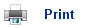# Reaction rates, temperature and catalysis

• As learned in previous modules, the rate of a reaction is increased by using a catalyst or increasing the temperature.
• The effect of using a catalyst and increasing the temperature can be explained using the collision theory.

## The collision theory of reactions

• The basic principle of the collision theory is that chemicals react when their particles collide with a certain minimum energy.
• Take the following reaction between nitrogen and hydrogen to produce ammonia:

N2(g) + 3H2(g)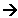2NH3(g)

• The collision theory states that H2 and N2 will react when they collide with enough energy. The more frequently they collide, the faster the reaction.
• If the pressure is increased, there will be more molecules of gas per unit volume; this will mean that there will be a greater chance of a successful collision; therefore the rate of the reaction is greater. This is one of the reasons why the Haber process uses high pressures.
• Increasing the temperature of the reaction gives the molecules more kinetic energy; this means that they will be moving around faster and will therefore be more likely to collide and successfully react. It is possible to calculate how much faster they move around, for the speed of the molecules is proportional to the square root of the temperature. So if the temperature of a reaction is increased 10K from 300K to 310K, then the speed of the molecules will increase by a factor of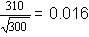; however the actual rate of the reaction doubles.
• This is related to the other part of the collision theory, which states that the particles must collide with a certain minimum energy (the activation energy).
• Unless they collide with this minimum amount of energy, they simply bounce off one another and remain unreacted: this happens to most of the molecules most of the time: at 300K only 1 in 1011 collisions of H2 and N2 is successful.

## The distribution of energies

• At any given temperature, the kinetic energies of the molecules in a substance are spread over a wide range of values; some will have very high kinetic energies and others will have lower kinetic energies.
• The distribution of kinetic energies can be shown in a Maxwell-Boltzmann Distribution:• As the temperature increases, the molecules move at higher speeds and therefore have higher kinetic energies.
• The Maxwell-Boltzmann distribution below shows the effect of increasing the temperature by 10 K: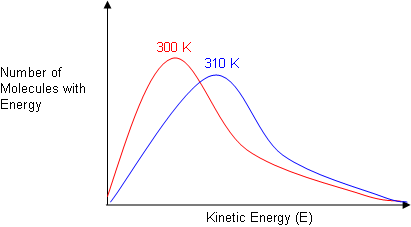• There is still a spread of kinetic energies; however, at the greater temperature, a greater proportion of the molecules have a higher energy.
• The effect of temperature on the rate of the reaction can be seen if the activation energy is added to the distribution: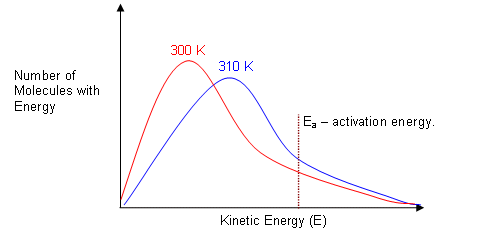• Only those molecules with energy greater than the activation energy are able to react; this is represented by the areas beneath the graphs to the right of the activation energy line.
• As you can see, at the higher temperature, a greater proportion of the molecules have energies above the activation energy; this means that more molecules will have enough energy to react, so the reaction goes faster.
• This can be summarised by saying that reactions go faster at higher temperatures because a larger proportion of the molecules have the minimum activation energy needed to react.
• Increasing the temperature does not make much difference to the individual molecule, but it does make a significant difference to the proportion of molecules with enough energy to react.

## Temperature and rate constants

• The rate constant for a reaction is given by the expression:

rate = k [A]a[B]b

• For many reactions, if the temperature of the reaction is increased by 10 K, the rate of the reaction will roughly double.
• Since the concentrations of the reactants are not changed, the equation above shows that it must be the rate constant, k, that changes with temperature.

## Catalysis

• Catalysts work by providing an alternative reaction pathway for the breaking and remaking of bonds. The activation energy for this new pathway is often less than the activation energy of the normal pathway.
• There are two different kinds of catalyst: heterogeneous catalysts are in a different state as the reactants, and homogenous catalysts are in the same state as the reactants.
• However the catalyst works, its effect is always the same. A catalyst lowers the activation energy needed for a reaction to occur; the molecular energy distribution remains the same, but as the activation energy is lower, a greater proportion of molecules will be above that energy and will therefore be able to react successfully.
• The heterogeneous catalyst in the Haber process is iron, it works as follows: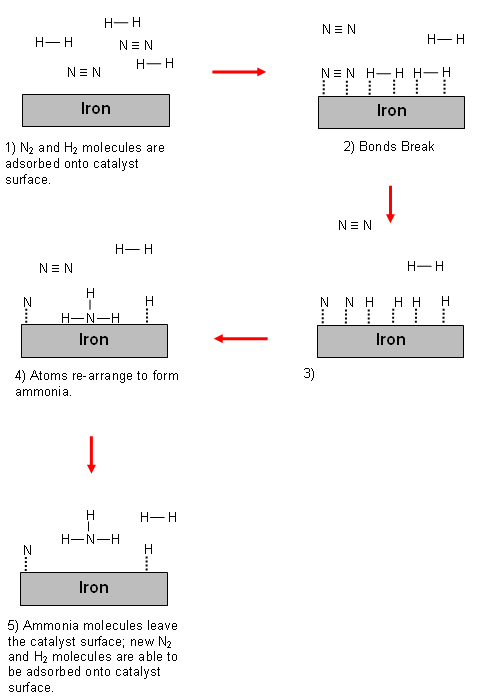• This process increases the rate of the reaction, because:
• Adhering to the catalyst surface uses electrons and so weakens the bonds in the reactants; this lowers the activation energy.
• There will be a greater concentration of reactants at the surface of the catalyst.
• The orientation of the reactants is correct for the reaction.

## Useful books for revision

Revise A2 Chemistry for Salters (OCR A Level Chemistry B)Salters (OCR) Revise A2 ChemistryHome